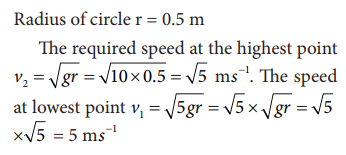Home | | Physics 11th std | Solved Example Problems for Motion in a vertical circle

# Solved Example Problems for Motion in a vertical circle

Physics : Work, Energy and Power : Motion in a vertical circle

Solved Example Problems for Motion in a vertical circle

### Example 4.17

Water in a bucket tied with rope is whirled around in a vertical circle of radius 0.5 m. Calculate the minimum velocity at the lowest point so that the water does not spill from it in the course of motion. (g = 10 ms-2)

### SolutionStudy Material, Lecturing Notes, Assignment, Reference, Wiki description explanation, brief detail
11th Physics : UNIT 4 : Work, Energy and Power : Solved Example Problems for Motion in a vertical circle |